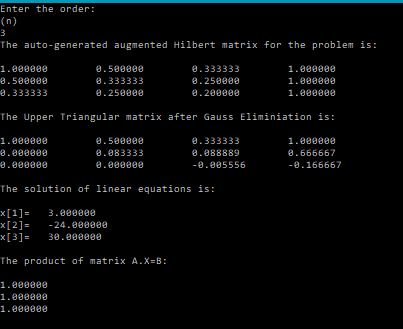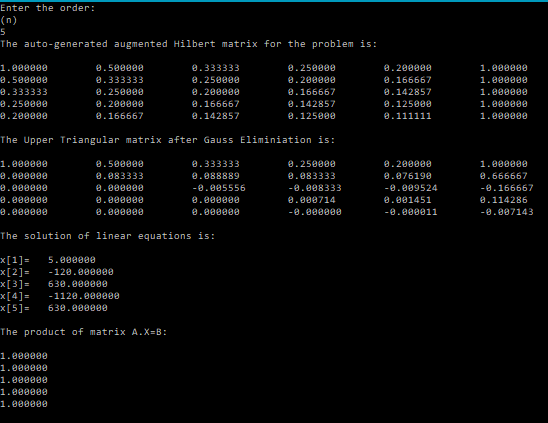# Gauss Elimination – EXERCISES [C Programming]

In this post we will be doing a few problems on Gauss-Elimination. These problems/exercises were given in my Numerical Analysis class.

These will help in polishing one’s skills in solving different kind of systems, and working with different kinds of matrices, and in the process bring out some inherent problems/intricacies with the Gaussian Elimination procedure.

### Exercise 1:

Solve a system of equations, given by:$HX=B$
where$H$ is a square Hilbert matrix whose elements are given as:$H_{ij}=\frac{1}{i+j-1}$
where$i,j=1,2,...,n$
and$B=[1,1,1,...,1]^T$

Notice how the results change when you change the precision from float to double.

### CODE:

/**gaussElim Exercise***/
#include<stdio.h>
#include<math.h>
/*******
Function that performs Gauss-Elimination and returns the Upper triangular matrix and solution of equations:
There are two options to do this in C.
1. Pass the augmented matrix (a) as the parameter, and calculate and store the upperTriangular(Gauss-Eliminated Matrix) in it.
2. Use malloc and make the function of pointer type and return the pointer.
This program uses the first option.
********/
void gaussEliminationLS(int m, int n, double a[m][n], double x[n-1]){
int i,j,k;
for(i=0;i<m-1;i++){
//Partial Pivoting
for(k=i+1;k<m;k++){
//If diagonal element(absolute vallue) is smaller than any of the terms below it
if(fabs(a[i][i])<fabs(a[k][i])){
//Swap the rows
for(j=0;j<n;j++){
double temp;
temp=a[i][j];
a[i][j]=a[k][j];
a[k][j]=temp;
}
}
}
//Begin Gauss Elimination
for(k=i+1;k<m;k++){
double  term=a[k][i]/ a[i][i];
for(j=0;j<n;j++){
a[k][j]=a[k][j]-term*a[i][j];
}
}

}
//Begin Back-substitution
for(i=m-1;i>=0;i--){
x[i]=a[i][n-1];
for(j=i+1;j<n-1;j++){
x[i]=x[i]-a[i][j]*x[j];
}
x[i]=x[i]/a[i][i];
}

}
/*******
Function that generates the Hilbert matrix
Parameters: order (n) ,matrix[n][n]
*******/
void genMatrix(int n, double matrix[n][n]){
int i,j;
//Initialize Coefficients
for(i=0;i<n;i++){
for(j=0;j<n;j++){
matrix[i][j]=(double)1.0/((i+1)+(j+1)-1);
}
}
}
/*******
Function that generates the Augmented Hilbert matrix
Parameters: order (n) ,matrix[n][n+1]
*******/
void genAugMatrix(int n, double matrix[n][n+1]){
int i,j;
//Initialize Coefficients
for(i=0;i<n;i++){
for(j=0;j<n;j++){
matrix[i][j]=(double)1.0/((i+1)+(j+1)-1);
}
}
//Initialize RHS part
for(i=0;i<n;i++){
matrix[i][n]=1;
}
}
/*******
Function that prints the elements of a matrix row-wise
Parameters: rows(m),columns(n),matrix[m][n]
*******/
void printMatrix(int m, int n, double matrix[m][n]){
int i,j;
for(i=0;i<m;i++){
for(j=0;j<n;j++){
printf("%lf\t",matrix[i][j]);
}
printf("\n");
}
}
/*******
Function that copies the elements of a matrix to another matrix
Parameters: rows(m),columns(n),matrix1[m][n] , matrix2[m][n]
*******/
void copyMatrix(int m, int n, double matrix1[m][n], double matrix2[m][n]){
int i,j;
for(i=0;i<m;i++){
for(j=0;j<n;j++){
matrix2[i][j]=matrix1[i][j];
}
}
}
/*******
Function that calculates the product of two matrices:
There are two options to do this in C.
1. Pass a matrix (prod) as the parameter, and calculate and store the product in it.
2. Use malloc and make the function of pointer type and return the pointer.
This program uses the first option.
********/
void matProduct(int m, int n, int n1,double a[m][n1], double b[n1][n], double prod[m][n]){
int i,j,k;
for(i=0;i<m;i++){
for(j=0;j<n;j++){
prod[i][j]=0;
for(k=0;k<n1;k++){
prod[i][j]=prod[i][j]+a[i][k]*b[k][j];
}
}
}
}

int main(){
int n,i,j;
printf("Enter the order:\n(n)\n");
scanf("%d",&n);
//Declare a matrix to store the augmented Hilbert matrix for the problem
double a[n][n+1];
//Declare another matrix to store the resultant matrix obtained after Gauss Elimination
double U[n][n+1];
//Declare an array to store the solution of equations
double x[n];
genAugMatrix(n,a);
printf("The auto-generated augmented Hilbert matrix for the problem is:\n\n");
printMatrix(n,n+1,a);
copyMatrix(n,n+1,a,U);
//Perform Gauss Elimination
gaussEliminationLS(n,n+1,U,x);
printf("\nThe Upper Triangular matrix after Gauss Eliminiation is:\n\n");
printMatrix(n,n+1,U);
printf("\nThe solution of linear equations is:\n\n");
for(i=0;i<n;i++){
printf("x[%d]=\t%lf\n",i+1,x[i]);
}
//Now we will verify if the answer is correct by multiplying X (solution) with the Hilbert matrix and see if we get 1.
double B[n]; //matrix to store product: A.X=B
//Declare a matrix to store the un-augmented(square) Hilbert matrix for the problem
double a1[n][n];
genMatrix(n,a1);
matProduct(n,1,n,a1,x,B);
//Print the product to verify
printf("\nThe product of matrix A.X=B:\n\n");
printMatrix(n,1,B);
}


### OUTPUT:### Android Apps:

I’ve also created a few Android apps that perform various matrix operations and can come in handy to those taking a course on Numerical Methods.